2018.10.16

## Potentially relevant bits of my story

• Very lazy software engineer; like to automate things
• Worked with some Expert Systems folks for a couple years at a place known for automated decision-making
• The inability of these systems to deal with uncertainty bothered me to no end
• Took time off to do Recurse Center and dive in
• Realized the majority of people trying to solve problems like this were actaully doing Bayesian modeling
• Joined Stan Core Development team

## How do we make decisions?

• Try to understand the state of the world
• Guess at the processes through which the world reacts to stimuli
• Associate utility scores with possible world states, and model relative chance
• Often, communication of findings is key

## Why is it hard to assign utilities to actions?

• Hard to represent the state of our knowledge about the world and how it changes
• We have uncertainty and ambiguity around even the most deterministic of processes
• And limited resources to discover the true process
• Many possible models for a process
• We will never know the True Model
• Nor the world’s True State

## Concrete example - mail delivery

• I get a few items of mail most days, but some days I get 0
• How often should I be experiencing no-mail days?
• I could use statistical analysis to make a case to a reporter that it is worth investigating
• …imagine that I care a lot about the mail

## Mail delivery uncertainty

• Mail is easy to measure, but the process that generates mail is incredibly complex
• For example, in a moment of weakness I used some expiring airline miles to subscribe to Vanity Fair
• No one has time to model my weaknesses
• Assume that the rate of mail creation is constant over time

## The Poisson distribution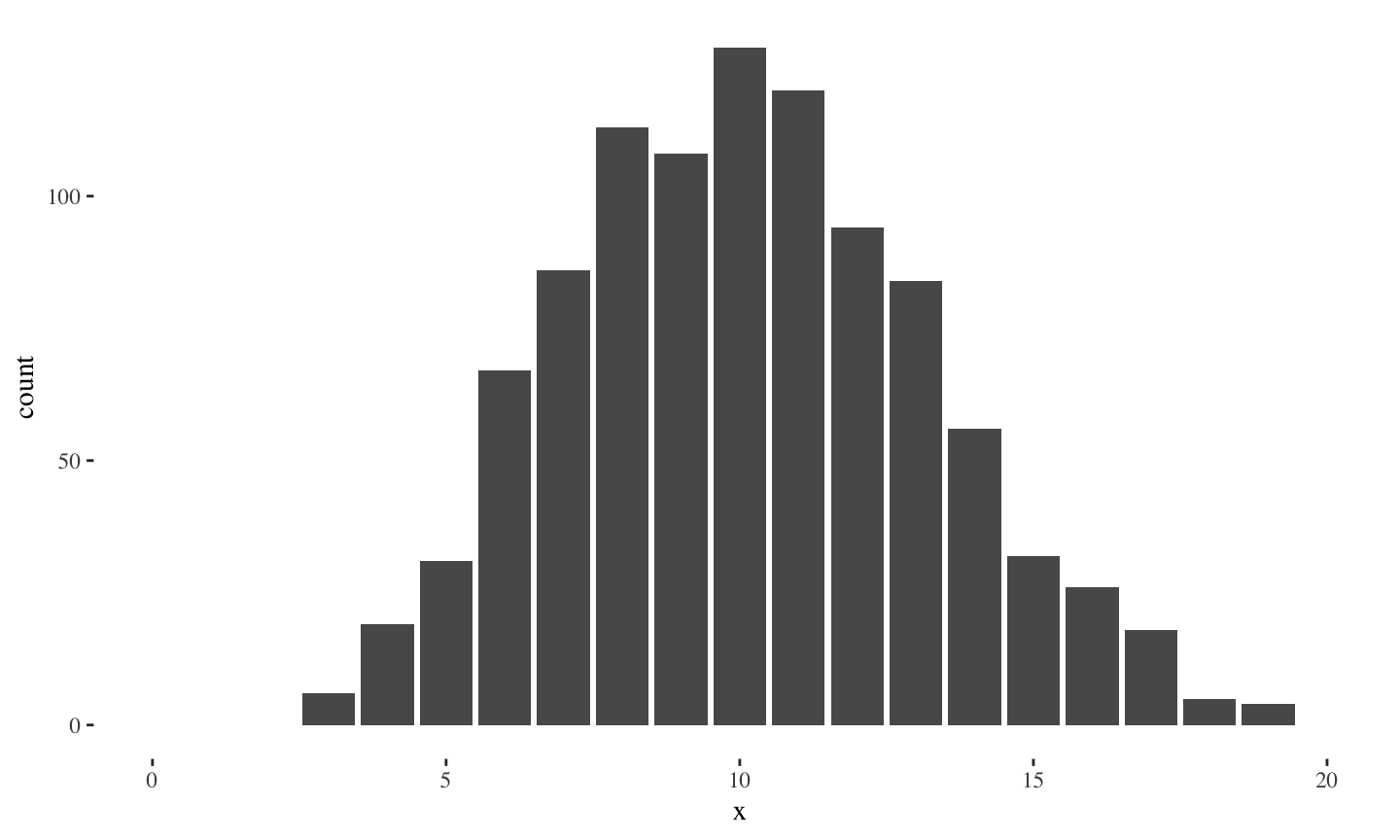## Modeling mail delivery with a Poisson

• A Poisson distribution captures much of the behavior we care about, but
• Exactly how well does it describe our data?
• Want to keep track of our uncertainty when making predictions or decisions
• We suspect someone is messing with our Poisson
• Visually explain to our reporter friend in terms they find compelling

## Frequentism, unfairly summarized

• Lots of totally valid frequentist math and procedures
• Typically quite difficult
• Oh, and make sure this list of assumptions all hold
• Alternatively, do a ton of math to prove asymptotic gaurantees specific to your model
• No parameter uncertainty, because probability only represents long-run frequency
• This actually matters when thinking about and communicating results
• “No” priors; incorporate minimal expert knowledge

## Machine learning, fairly summarized

• We have computers now, calculus can GTFO
• fair enough
• Great success with algorithms that have only later had statistical interpretations ascribed to them
• Progress = predictions 0.01% better than the last paper according to cross validation
• Let’s ignore that the noise around cross-validated accuracy scores is much higher than 0.01%
• Why would we need to interpret the model if its predictions are accurate?

## What does Bayesianism preach?

• Philosophically, inference without a prior is impossible
• In frequentism it’s buried in an assumption
• In ML you are not really sure if there is a deeper meaning under the code in the first place, but there is some implied prior
• Retain a distribution (as opposed to point estimates like the mean) as long as possible before making a decision
• We also (only recently?) have computers!
• We can actually express an accurate, bespoke model for our data generating process without worrying about an analytic integral
• Construct many models
• Always Be Graphing

## What are we modeling?

• “If we knew our parameter values $$\theta$$, how would data $$y$$ be generated?” $\pi(y \, | \, \theta)$
• But we want to learn what our data implies about $$\theta$$
•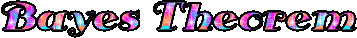$\pi(\theta \, | \, y) = \frac{\pi(y \, | \, \theta) \pi(\theta)}{\pi(y)}$
• In our case, $$\theta$$ might be the average amount of mail we get on any given day, or the percentage of days the carrier skips

## Practical modeling with Stan

• Stan is an imperative probabilistic programming language
• in the vein of BUGS, but can do arbitrary computation
• A Stan program defines a probability model
• declares data inputs and parameter variables
• defines a log posterior density function
• Stan comes with a few inference algorithms
• Hamiltonian Monte Carlo for full Bayesian inference
• Variational Inference
• penalized Maximum Likelihood Estimation and optimization

## Why choose Stan?

• Expressive
• Stan is a full imperative programming language
• Easier to communicate models
• Robust
• usually works; signals when it doesn’t
• Efficient
• effective sample size per unit time winner
• 3 forms of parallelism coming out this year (CPU, GPU, cluster)
• Great documentation, case studies, and community!

## Anatomy of a Stan model

• data and parameters just declare inputs to a density function
• model block defines that function and, given some data and parameters, returns a scalar real value density of the posterior at that location in parameter space
• This defines $$\pi(y \, | \, \theta)$$ from a few slides ago
• Stan provides inference mechanisms to learn $$\pi(\theta \, | \, y)$$ from a model’s $$\pi(y \, | \, \theta)$$

## Normal mail delivery Stan model

data {
int<lower=0> num_days;
int<lower=0> mail[num_days];
}
parameters {
real<lower=0> avg_mail_per_day;
}
model {
avg_mail_per_day ~ normal(0, 5);
mail ~ poisson(avg_mail_per_day);
}

## Fitting a Stan model with PyStan

import pystan, numpy as np
data = dict(mail = np.array(r["mail"], dtype="int"), num_days=int(r["num_days"]))
model = pystan.StanModel("usps1.stan")
fit = model.sampling(data)
4 chains, each with iter=2000; warmup=1000; thin=1;
post-warmup draws per chain=1000, total post-warmup draws=4000.

mean se_mean     sd   2.5%      50%  97.5%  n_eff   Rhat
avg_mail_per_day   4.96  2.3e-3   0.08    4.8    4.96   5.11   1316    1.0

For each parameter, n_eff is a crude measure of effective sample size,
and Rhat is the potential scale reduction factor on split chains (at
convergence, Rhat=1).

## Plotting the fit

from matplotlib import pyplot as plt
plt.hist(fit.extract()["avg_mail_per_day"], bins=20)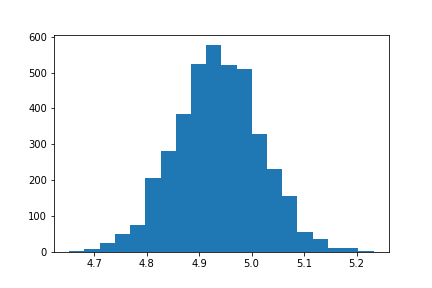## Visually communicating model fit

from matplotlib import pyplot as plt
mail_rate = fit.extract()["avg_mail_per_day"] # 4000 posterior samples for this param
fake_data = np.random.poisson(mail_rate) #4000 fake data simulations
plt.hist([fake_data, data["mail"]], bins=15, density=True, color=["red", "blue"])
• Posterior Predictive Check (PPC) plot
• Posterior distribution is a distribution over parameter space incorporating what we’ve learned about our parameters
• Simulate data based on the posterior and plot it against the actual observed data

## Visually communicating model fit

from matplotlib import pyplot as plt
mail_rate = fit.extract()["avg_mail_per_day"] # 4000 posterior samples for this param
fake_data = np.random.poisson(mail_rate) #4000 fake data simulations
plt.hist([fake_data, data["mail"]], bins=15, density=True, color=["red", "blue"])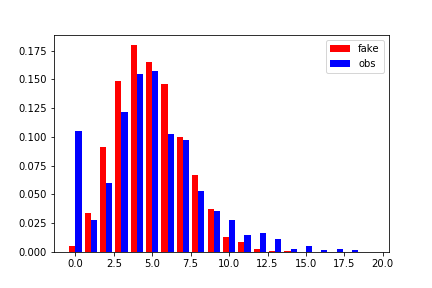## Add skip_percentage to model skipped days

data {
int<lower=0> num_days;
int<lower=0> mail[num_days];
}
parameters {
real<lower=0> avg_mail_per_day;
real<lower=0, upper=1> skip_percentage;
}
model {
avg_mail_per_day ~ normal(0, 5);
for (day in 1:num_days) {
real without_skipping_density = poisson_lpmf(mail[day] | avg_mail_per_day);
if (mail[day] == 0)
target += log_mix(skip_percentage, 0, without_skipping_density);
else
target += without_skipping_density;
}
}

## Fit model with skip_percentage

Inference for Stan model: anon_model_7abb4b18761050015bf05aee642e2a30.
4 chains, each with iter=2000; warmup=1000; thin=1;
post-warmup draws per chain=1000, total post-warmup draws=4000.

mean se_mean     sd   2.5%    50%  97.5%  n_eff   Rhat
avg_mail_per_day   5.77  2.0e-3   0.09   5.58   5.76   5.96   2328    1.0
skip_percentage    0.99  2.2e-4   0.01   0.96   0.99    1.0   2791    1.0
lp__              -1599    0.03   1.06  -1602  -1598  -1598   1336    1.0

Samples were drawn using NUTS at Tue Oct 16 22:41:45 2018.
For each parameter, n_eff is a crude measure of effective sample size,
and Rhat is the potential scale reduction factor on split chains (at
convergence, Rhat=1).

## Simulate data implied by parameter draws

mail_rate15 = fit15.extract()["avg_mail_per_day"]
skip_percentage = fit15.extract()["skip_percentage"]
fake_data15 = np.zeros(len(mail_rate))
for i in range(len(mail_rate15)):
todays_mail =  np.random.poisson(mail_rate15[i])
if np.random.binomial(1, skip_percentage[i]):
fake_data15[i] = 0
else:
fake_data15[i] = todays_mail

## Visually compare simulations with observed data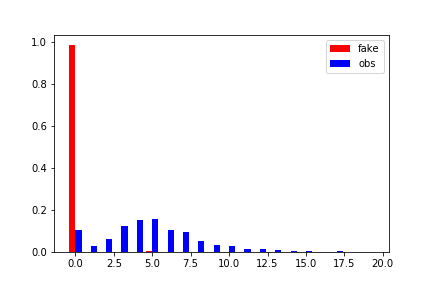## Visually compare simulations with observed data## Fix our math; intro log scales

parameters {
real<lower=0> avg_mail_per_day;
real<lower=0, upper=1> skip_percentage;
}
model {
avg_mail_per_day ~ normal(0, 5);
for (day in 1:num_days) {
real without_skipping_density = poisson_lpmf(mail[day] | avg_mail_per_day);
if (mail[day] == 0)
target += log_mix(skip_percentage, 0, without_skipping_density);
else
target += without_skipping_density
+ bernoulli_lpmf(0 | skip_percentage);
}
}

## Fixed fit

Inference for Stan model: anon_model_387876dd9af8a07353d37466d2bde6fb.
4 chains, each with iter=2000; warmup=1000; thin=1;
post-warmup draws per chain=1000, total post-warmup draws=4000.

mean se_mean     sd    25%    50%  97.5%  n_eff   Rhat
avg_mail_per_day   5.52  1.6e-3   0.09   5.46   5.52    5.7   3350    1.0
skip_percentage     0.1  2.0e-4   0.01    0.1    0.1   0.13   3072    1.0
lp__              -1821    0.02   0.97  -1821  -1821  -1820   1884    1.0

Samples were drawn using NUTS at Sat Oct 13 13:45:07 2018.
For each parameter, n_eff is a crude measure of effective sample size,
and Rhat is the potential scale reduction factor on split chains (at
convergence, Rhat=1).

## PPC plot for corrected model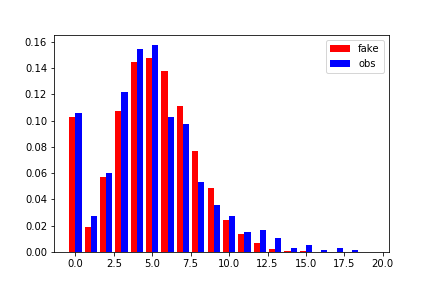## PPC plot for corrected model